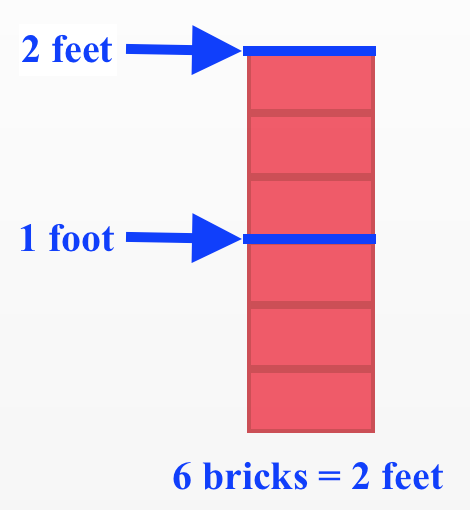### Home > MC1 > Chapter 1 > Lesson 1.1.5 > Problem1-46

1-46.

A stack of six bricks is two feet high. Use that information to answer the questions below.

1. How many bricks are in a stack $20$ feet high?

Use the diagram below for help.($10$ two-foot stacks)($6$ bricks per two-foot stack) $=60$ bricks

2. How high is a stack of $20$ bricks?

In part (a), you found that $60$ bricks make a stack $20$ feet high. Since $60=(3)(20)$, a stack of $20$ bricks should be one third the height of a stack of $60$ bricks.